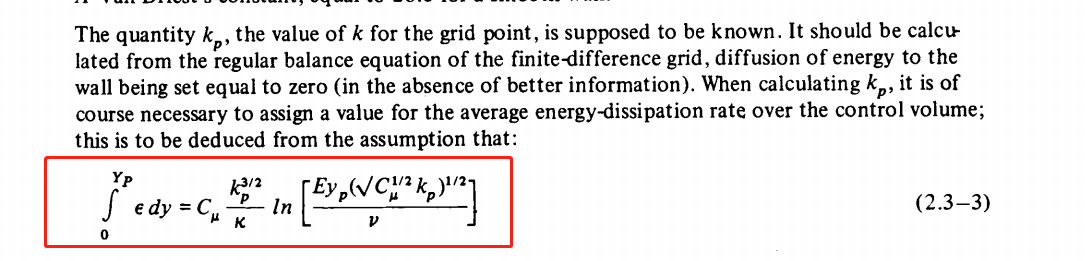# Code production of k ?

• The production of kinetic energy near wall cell is referred by which paper?

G0[celli] +=
w
*(nutw[facei] + nuw[facei])
*Cmu25*sqrt(k[celli])
/(kappa_*y[facei]);


I found Spalding's formula is not the same as above (Pk =Cmu^0.25Utau^2U/y). Anybody knows this issue?

• @东岳 Many thanks. In fact, I understood this procedure. One confusion is that the formula implemented in openfoam is not consistent with that mentioned in the Spalding' paper.• @东岳 Many thanks. In fact, I understood this procedure. One confusion is that the formula implemented in openfoam is not consistent with that mentioned in the Spalding' paper.Nobody is interested in this issue?

CFD中文网 2016 - 2020 | 京ICP备15017992号-2